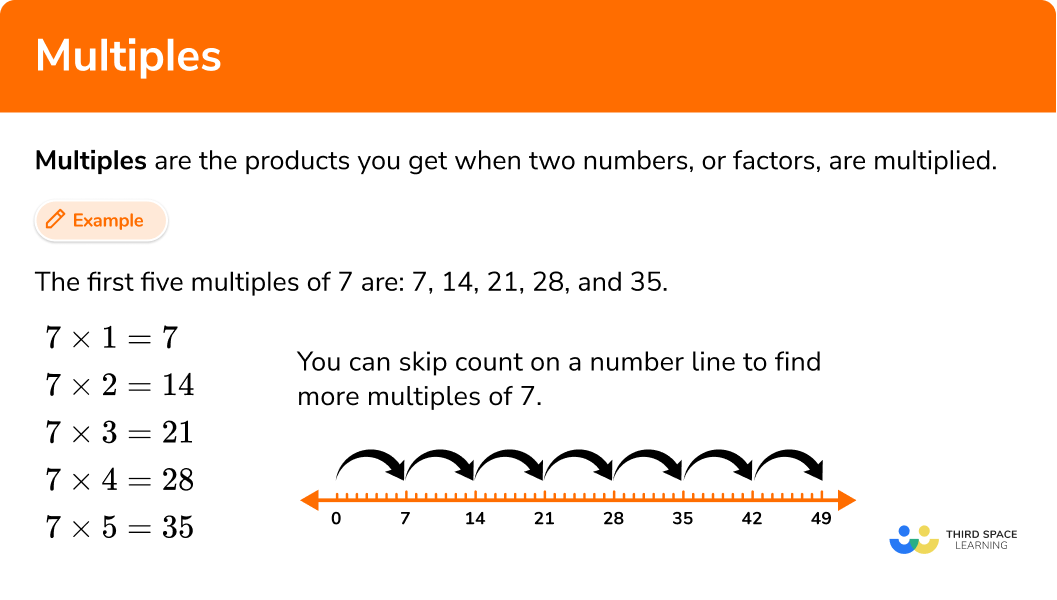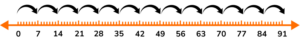# Multiples

Here you will learn about multiples, including how to calculate multiples of a number and solve problems using knowledge of multiples.

Students will first learn about multiples as part of operations and algebraic thinking in elementary school.

## What are multiples?

Multiples are the products you get when two numbers, or factors, are multiplied.

For example, the first five multiples of 7 are 7 , 14 , 21 , 28 , and 35 .

You can skip count on a number line to find multiples of 7 .

The number line shows the first 7 multiples of 7 , but you could keep skipping counting to find more multiples.

To make them easier to find, multiples of the numbers 1 to 9 have patterns. They can help you decide if a given number is a multiple of 1 to 9 .

NumberMultiplesPattern
1 1, 2, 3, 4, 5, 6, 7, 8, 9, 10…

every number is a multiple of 1

2 2, 4, 6, 8, 10, 12, 14, 16, 18…

the last digit is an even number

3 3, 6, 9, 12, 15, 18, 21, 24…

the sum of the digits is divisible by three

4 4, 8, 12, 16, 20, 24, 28, 32…

the number is double of a multiple of \bf{2}

5 5, 10, 15, 20, 25, 30, 35…

the last digit is zero or five

6 6, 12, 18, 24, 30, 36, 42, 48…

the number is divisible by both two and three

7 7, 14, 21, 28, 35, 42, 49, 56…

*No divisibility pattern

8 8, 16, 24, 32, 40, 48, 56…

the number is double a multiple of \bf{4}

9 9, 18, 27, 36, 45, 54, 63, 72…

the sum of the digits is divisible by nine

10 10, 20, 30, 40, 50, 60…

the last digit is zero

### What are multiples?## Common Core State Standards

How does this relate to 4th grade math?

• 4th Grade: Operations and Algebraic Thinking (4.OA.B.4)
Determine whether a given whole number in the range 1 to 100 is a multiple of a given one-digit number.

## How to identify multiples

In order to find if a number is a multiple of another number:

1. Think about the pattern for the multiples of the number.
2. Decide if the number is a multiple.

## Multiples examples

### Example 1: decide whether a number is a multiple

Is 76 a multiple of 6 ?

1. Think about the pattern for the multiples of the number.

Start a list of multiples for 6 :

6 , 12 , 18 , 24 , 30

Notice that the multiples of 6 are also multiples of 2 and 3 .

This pattern is true for all multiples of 6 .

2Decide if the number is a multiple.

✔ The last digit (6) is even, so it is a multiple of 2 .

✘ The sum of the digits (7 + 6 = 13) , so it is not a multiple of 3 .

76 is NOT a multiple of 6 because it is NOT a multiple of 3 .

Skip counting on a number line shows that 76 is NOT a multiple of 6 .

### Example 2: decide whether a number is a multiple

Is 65 a multiple of 5 ?

Start a list of multiples for 5 :

5 , 10 , 15 , 20 , 25

Notice that they end in either a 5 or a 0 .

This pattern is true for all multiples of 5 .

65 is a multiple of 5 because it ends in a 5 .

Skip counting on a number line shows that 65 is the 13^{th} multiple of 5 .

### Example 3: decide whether a number is a multiple

Is 66 a multiple of 4 ?

Start a list of multiples for 4 :

4 , 8 , 12 , 16 , 20

Notice that they are all doubles of a multiple of 2 .

This pattern is true for all multiples of 4 .

66 is the double of 33 \rightarrow 33 \times 2=66 , but 33 is not a multiple of 2 .

66 is not a multiple of 4 because it is not a double of a multiple of 2 .

Skip counting on a number line shows that 66 is NOT a multiple of 4 .

### Example 4: decide whether a number is a multiple

Is 72 a multiple of 9 ?

Start a list of multiples for 9 :

9 , 18 , 27 , 36 , 45

Notice that the sum of the digits is divisible by 9 .

This pattern is true for all multiples of 9 .

72 is a multiple of 9 , because the sum of the digits (7+2=9) is divisible by 9 .

Skip counting on a number line shows that 72 is the eighth multiple of 9 .

### Example 5: decide whether a number is a multiple

Is 42 a multiple of 3 ?

Start a list of multiples for 3 :

3 , 6 , 9 , 12 , 15

Notice that the sum of the digits is divisible by 3 .

This pattern is true for all multiples of 3 .

42 is a multiple of 3 , because the sum of the digits (4+2=6) is divisible by 3 .

Skip counting on a number line shows that 42 is the 14^{th} multiple of 3 .

### Example 6: decide whether a number is a multiple

An elementary school is performing a play. They want to put out 88 chairs for the audience. If they want the same amount of chairs in each row, should they do rows of 6 , 8 or 10 ?

Multiples of 6 : the sum of the digits is divisible by three.

Multiples of 8 : the number is double a multiple of 4 .

Multiples of 10 : the last digit is zero.

88 is NOT a multiple of 6 , because the sum of the digits (8 + 8 = 16) is NOT divisible by 3 .

88 is a multiple of 8 , because it is double a multiple of 4 \rightarrow 2 \times 44=88 .

The school should put the 88 chairs in rows of 8 .

### Teaching tips for multiples

• Use a multiplication table so that students can see examples of multiples and look for patterns.

• While students will need to remember the multiple patterns to solve quickly, avoid just using math worksheets. Help students deepen their understanding and likelihood of remembering by also doing hands-on activities. For example, give students 52 counters and ask them to make as many arrays as possible to find out what factors 52 is a multiple of.

• Play games regularly to help students memorize larger multiples. For example, have students count starting from 1 (the first student says 1 , the second says 2 , the third says 3 and so on), but challenge them to use a different word for a certain multiple each time. So if they were playing with multiples of 7 , whenever a student got to a multiple of 7 , they would say “cookie” instead of the multiple.

### Easy mistakes to make

• Confusing the terms multiples and factors
Factors and multiples are easily mixed up. Remember multiples are the numbers in the middle of the multiplication table, whereas factors are the numbers around the outside (the numbers being multiplied).

• Forgetting the number itself as a multiple
All numbers are a multiple of themselves.
For example, the multiples of 6 are: 6 , 12 , 18 , 24 and so on and so 6 is a multiple of itself.

### Practice multiples questions

1. Which number is a multiple of 2 ?

54897791For multiples of 2 , the last digit is an even number. 54 ends in an even number and 27 \times 2=54 , so 54 is a multiple of 2 .

2. Which number is a multiple of 7 ?

99839174Using a number line to skip count by 7 , shows that 91 is a multiple of 7 .3. Which number is NOT a multiple of 3 ?

66433951For multiples of 3 , the sum of the digits is divisble by three. 4+3=7 is not divisible by 3 , so 43 is NOT a multiple of 3 .

4. Which number is NOT a multiple of 4 ?

88682858For multiples of 4 , the number is double a multiple of 2 . 58 is the double of 29 , so 58 is NOT a multiple of 4 .

5. A grocery store has 78 boxes of cereal. They want to put the same number of boxes on each shelf. How many boxes should they put on each shelf?

4 boxes5 boxes6 boxes7 boxesFor multiples of 6 , the number is divisible by both two and three. 78 is a multiple of 2 , because it ends in an even number (8) .
78 is a multiple of 3 , because 7 + 8 = 15 and 15 \div 3 = 5 .

So 78 is a multiple of 6 \rightarrow 6 \times 13=78
The grocery store can put 6 boxes on each shelf.

## Multiples FAQs

What is the difference between multiples and times tables?

Times tables are a list of multiplication equations for a certain number and include both factors and multiples. Multiples are the answers (products) in times tables.

Can I use division to find multiples?

Yes, if a number divides another number without a remainder, it’s a multiple. For example, in the question “is 72 a multiple of 2 ?” you can solve 72 \div 2=36 . There are no remainders, so it is a multiple.

Do negative numbers have multiples?

All positive and negative numbers have multiples. However, in 4th grade math you only work with positive numbers.

Do prime numbers have multiples?

Prime numbers have an infinite amount of multiples but only 2 factors.

## Still stuck?

At Third Space Learning, we specialize in helping teachers and school leaders to provide personalized math support for more of their students through high-quality, online one-on-one math tutoring delivered by subject experts.

Each week, our tutors support thousands of students who are at risk of not meeting their grade-level expectations, and help accelerate their progress and boost their confidence.